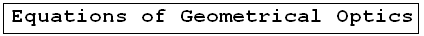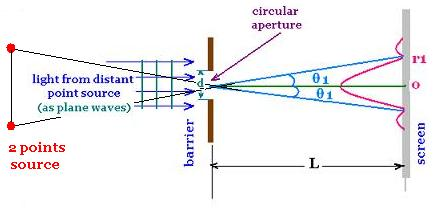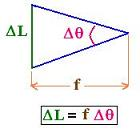ÂGeometrical Optics: Geometrical Optics & Physics Optics. a Huygens Principle: Wavefronts. a Reflection: The first law of Geometrical Optics. a Refraction: The second law of Geometrical Optics: Snell's law. a Critical angle: Internal total reflection. a images formed by reflection Spherical concave mirrors. Mirror equation. a images formed by refraction Spherical convex mirrors .. a Fermat's principle: Fermat's principle for reflection and refraction.. a lenses: Thin lenses and Lens-makers' equation .. a prism: Prism, colors minimum deviation .. a Human eye: near point, far point, nearsightedness, farsightedness.. a Microscope: Microscope: simple, compound, and magnification.. a Telescope: Telescope: magnification.. a Resolution: optical microscope, electron microscope .. a dispersion: dispersion, more about the refractive index, and colors of a prism .. a parallel sheet: deviation by a parallel sheet .. a thin films : thin films and Newton's rings.. a Newton's rings: thin films and Newton's rings.. a Descartes rainbow: Primary and secondary rainbows .. a Some applications More fun with Optics .. a Optics calculator: Optics calculator: all the related calculations .. a home The fundamental, and just this .. a scientificSentence a __________________ a

Geometrical Optics
Optics - Â  Â  Geometrical Optics..

### Optical instrument Resolution :

#### 1. Definition:

Theresolution is an important property of any optical instrument, such as a microscope, a telescope, a camera, or an eye.

The resolution of a certain optical device is its ability to show two points apart as separate points. In other words, it is the measure of its ability to produce separate images of two adjacent points objects. It is also called a resolving power of the device. For example, if the resolution of a device is equal to Re, and the two points are separated by S. If S < Re then the two points will be blended and seen blured, if S > Re, then the two points will be seen separated as really they are.

#### 2. Expression of the resolution

We have seen, with the Fraunhofer diffraction by a circular aperture, the expression of the angular position Î¸1 related to Airy disk (the first dark ring surrounding the bright central maximum) is

sin Î¸1 = 1.22 Î»/d
Î» is the wavelength of the used light in the device,
and d is the diameter of the circular aperture of the device.

Î¸1 is small, so: Î¸1 = 1.22 Î»/dWe have:

r1 = L Î¸1 = 1.22 Î»L/d

The central maximum is more spread out for longer wavelengths and for smaller apertures.

For an optical instrument, the circular aperture is the lens (for a microscope, a camera) or the entrance mirror (for a telescope). The screen is an eye or detector.

Two points source of light (as stars for a telescope) produce two images that are diffraction patterns with no interference seen at the distance L (>> d) from the circular aperture.

The angle separation of the two points source from the eye (or detector) must allow to show two points, not one. For this, the resolution is defined by a criterion. We use the Rayleigh criterion. According to this criterion, two images are just resolved if the center of the central maximum of one pattern falls on the first dark ring of the other. Therefore,

Two point objects separated by an angle ÎÎ¸ are clearly resolved when ÎÎ¸ > ÎÎ¸R

ÎÎ¸R is the limiting angle of resolution for an optical instrument with an aperture diameter d receiving a light of wavelength Î». We have:

ÎÎ¸R = 1.22 Î»/dFor a microscope, the distance L is close to the focal length f of the objective, the spatial resolution is:
r1 = Î¸1 = 1.22 Î»L/d, or

Îl = 1.22 f Î»/d

For a telescope, the aperture diameter "D" of its objective is large (about 5 meters), then we can take 1.22 = 1, so
ÎÎ¸R = Î»/D

#### 3. Electronic microscope:

Instead of using light, we use electrons. We know that they behave as waves according to De Broglie formula:

Î» = h/p
h is the Planck's constant, p the magnitude of the linear momentum, and Î» the associated wavelength for the electron.

If E is the kinetic energy of the electron, E = mv2/2
Then
p = mv = m (2E/m)1/2 = (2mE)1/2 Using the De Broglie relationship p = h/Î» yields:
Î» = h/p = h/(2mE)1/2
Therefore, the angular resolution power is:
ÎÎ¸R = 1.22 Î»/d = 1.22 h/d(2mE)1/2

h (Planck's constant) = 6.63 × 10-34 m2 kg/s
m (electron mass) = 9.11 × 10-31 kilograms
1 eV (electron volt) = 1.60 x 10-19 joules
1 cm = 1.0 x 10-2 m
Then:
ÎÎ¸R = 1.22 h/d(2mE)1/2 = 1.22 x 6.63 × 10-34/(2m)1/2 d(E)1/2
= 1.22 x 6.63 × 10-34/(2 x 9.11 × 10-31 x 1.60 x 10-19)1/2 x 1.0 x 10-2 d(E)1/2 = 1.5 x 10-9/d E1/2

ÎÎ¸R = 1.5 x 10-9/dE1/2
d in m, and E in eV

ÎÎ¸R = 1.5 x 10-7/dE1/2
d in cm, and E in eV

#### 4. Comparison: ÎÎ¸R(light) and ÎÎ¸R(electron)

If E = 1.0 eV , and d = 1.0 cm
ÎÎ¸R = 1.5 x 10-7 = 0.15 x 10-6 rad = 0.15 Âµn;rad.

If we consider a yellow light with a wavelength of 580 nm, the resolution will be:
ÎÎ¸R = 1.22 x 580x -9/1.0 x -2 708 x -7 rad = 71 Âµrad

The limiting resolution of an electron microscope is much higher than that of an optical microscope

##### Remark:

For an electron, the wavelength is Î» = h/(2mE)1/2 =
6.63 × 10-34/(2x 9.11 × 10-31x 1.60 x 10-19E)1/2 = 1.23 x 10-9 /E1/2
Therefore:

Î»electron = 1.23 nm/E1/2
E in eV

Â

 @import url(http://www.google.com/cse/api/branding.css);Custom Search Â© 2009. The scientificsentence . All rights reserved.